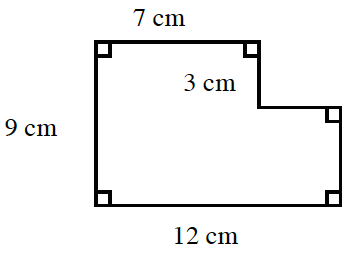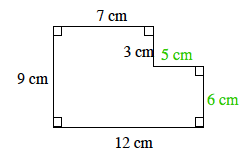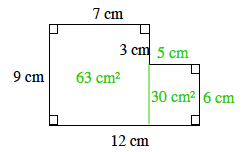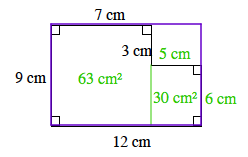### Home > CC1MN > Chapter 7 Unit 7 > Lesson CC1: 7.2.2 > Problem7-54

7-54.

Find the area of the shape at right in at least two ways.Label each remaining side using centimeters.

Our first method of finding the area is to divide the shape into smaller rectangles and label their areas.

$(9)(7) + (5)(6) = 63 + 30 \\= 93 \text{ square centimeters}$

Another method for finding the area is to subtract the area of the rectangle in the upper-right corner from the area of the purple rectangle. Show that this method gives the same answer as Method 1.